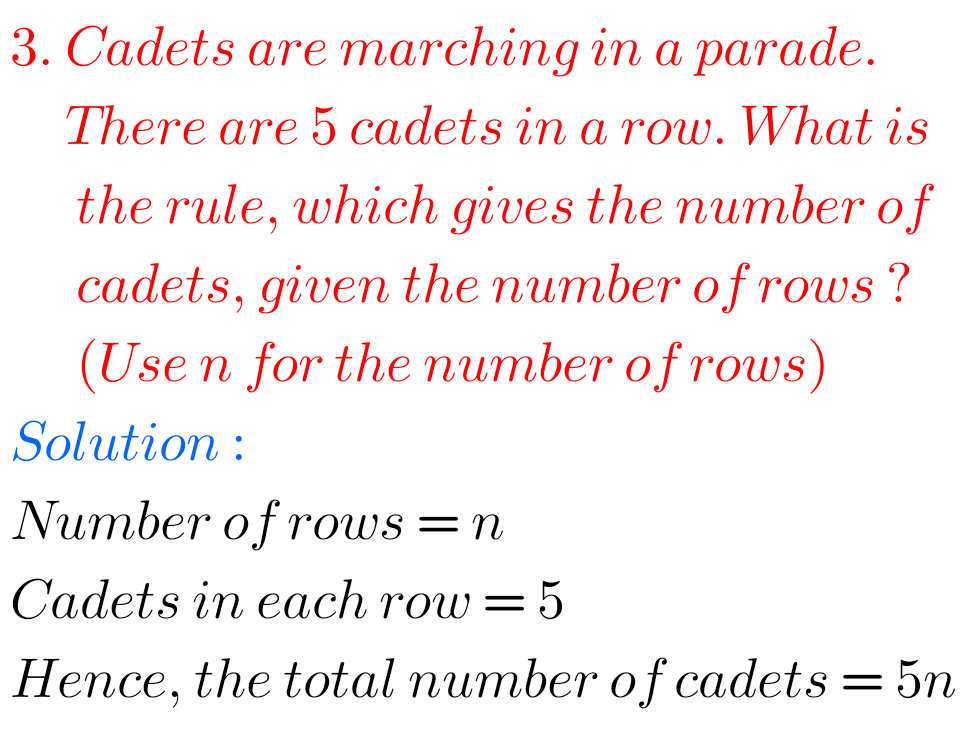## Ncert solutions for maths class 6 Algebra exercise 11.1 chapter 11

Maths class 6 chapter 11 exercise 11.1 Ncert solutions Class 6 chapter 11 Algebra exercise 11.1 solutions  Maths Ncert textbook are given. Study the textbook lesson Algebra very well Observe the example problems and solutions given in the solutions. Observe the solutions and try them in your own method. You can also see Ncert Maths …